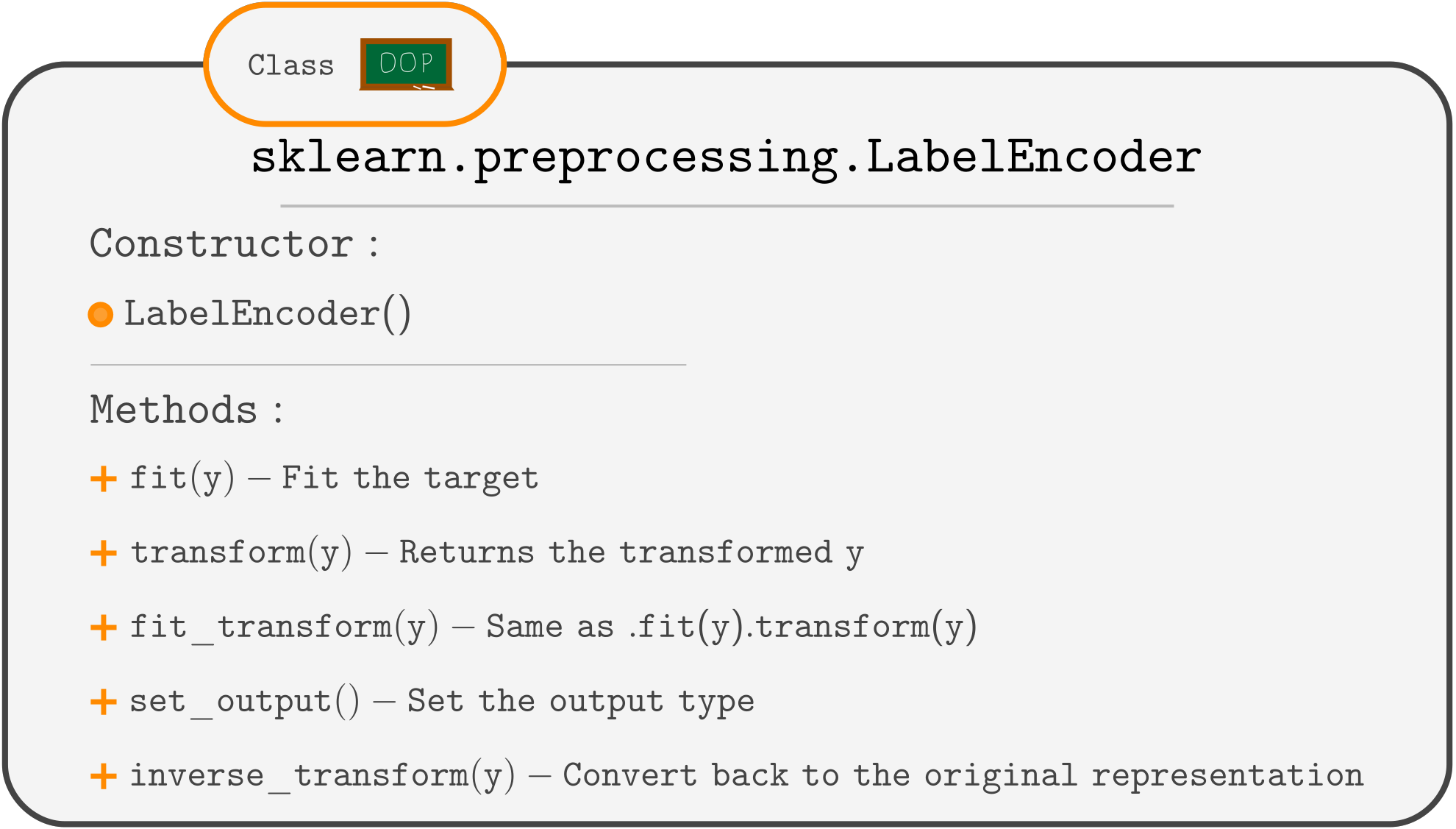Course Content

ML Introduction with scikit-learn

##Final Estimator Challenge

Now let's build a proper pipeline with the final estimator. As a result, we will get a trained prediction pipeline that can be used for predicting new instances simply by calling the `.predict()` method.
To train a predictor (model), you need `y` to be encoded. This is done separately from the Pipeline we build for `X`.
Remember that `LabelEncoder` is used for encoding the target.You have the same Penguins dataset.
The task is to build a pipeline with `KNeighborsClassifier` as a final estimator, train it, and predict values for the `X` itself.
Since the predictions will be encoded (0, 1, or 2), we will use the `.inverse_transform()` method of `LabelEncoder` to get the predictions back to 'Adelie', 'Chinstrap', or 'Gentoo'.

1. Encode the `y` variable using the `LabelEncoder`.
2. Make a pipeline containing `ct`, `SimpleImputer`, `StandardScaler`, and `KNeighborsClassifier` (in that order).
3. Train the `pipe` object.